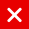# IOTA Tutorial

## Trit and Tryte

In this video series different topics will be explained which will help you to understand IOTA.
It is recommended to watch each video sequentially as I may refer to certain IOTA topics explained earlier.

The trinary numeral system has two types:
The balanced trinary system in which a trit has the values -1, 0 and 1.
The unbalanced trinary system in which a trit has the values 0, 1 and 2.
In this presentation I will only focus on the balanced trinary system.
Trit means Trinary Digit, analogous to bit and has the following values: -1, 0 and 1.
Tryte means Trinary Byte, analogous to byte.
A tryte consists of 3 trits.

1 byte = 2^8 = 256 combinations
1 tryte = 3 trits = 3^3 = 27 combinations
5 trits = 3^5 = 243 combinations
5 trits is NOT equal to 1 byte

Convert tryte -1, 1, 0 to integer:
-1 x 3^0 + 1 x 3^1 + 0 x 3^2 = 2
Convert tryte 1, -1, 1 to integer:
1 x 3^0 + -1 x 3^1 + 1 x 3^2 = 7

What is the maximum value a tryte can have (not the number of combinations)?
If you thought 3^3 – 1 = 26 you are thinking in the binary system.
If you have 2 bits in a binary system, you have the following combinations:
00 = 0x2^1 + 0x2^0 = 0
01 = 0x2^1 + 1×2^0 = 1
10 = 1×2^1 + 0x2^0 = 2
11 = 1×2^1 + 1×2^0 = 3
Max value = 2^2 – 1

If you have 2 trits in a balanced trinary system, you have the following combinations:
0, 0 = 0x3^0 + 0x3^1 = 0
0, 1 = 0x3^0 + 1×3^1 = 3
0,-1 = 0x3^0 + -1×3^1 = -3
1, 0 = 1×3^0 + 0x3^1 = 1
1, 1 = 1×3^0 + 1×3^1 = 4
1,-1 = 1×3^0 + -1×3^1 = -2
-1, 0 = -1×3^0 + 0x3^1 = -1
-1, 1 = -1×3^0 + 1×3^1 = 2
-1,-1 = -1×3^0 + -1×3^1 = -4

The values in the trinary system are balanced around zero:
-4, -3, -2, -1, 0, 1, 2, 3, 4
Max value = (3^2 – 1) / 2

A tryte has 3 trits, so the maximum value will be (3^3 -1) / 2 = 13 and it has 3^3 = 27 combinations.
A tryte will have the following values: -13, -12, …-2, -1, 0, 1, 2,…12, 13
Convert the following two trytes -1, -1, -1, 1, 0, 0 to an integer:
-1 x 3^0 + -1 x 3^1 + -1 x 3^2 + 1 x 3^3 + 0 x 3^4 + 0 x 3^5
-13 + 27 = 14

IOTA uses the balanced trinary system
To make the trytes more human readable the IOTA development team created the tryte alphabet:
9ABCDEFGHIJKLMNOPQRSTUVWXYZ
The tryte alphabet consists of 26 letters of the latin alphabet plus the number 9.
The tryte alphabet has a total of 27 characters.
Because 1 tryte has 3^3 = 27 combinations, each tryte can be represented by a character in the tryte alphabet.

Tryte alphabet:
Tryte Dec Char
0, 0, 0 0 9
1, 0, 0 1 A
-1, 1, 0 2 B
0, 1, 0 3 C
1, 1, 0 4 D
-1,-1, 1 5 E
0,-1, 1 6 F
1,-1, 1 7 G
-1, 0, 1 8 H
0, 0, 1 9 I
1, 0, 1 10 J
-1, 1, 1 11 K
0, 1, 1 12 L
1, 1, 1 13 M
-1,-1,-1 -13 N
0,-1,-1 -12 O
1,-1,-1 -11 P
-1, 0,-1 -10 Q
0, 0,-1 -9 R
1, 0,-1 -8 S
-1, 1,-1 -7 T
0, 1,-1 -6 U
1, 1,-1 -5 V
-1,-1, 0 -4 W
0,-1, 0 -3 X
1,-1, 0 -2 Y
-1, 0, 0 -1 Z

IOTA seeds, addresses, hashes, etc are trytes which are represented by characters from the tryte alphabet.
For example the integer 14, converted into trytes: -1, -1, -1, 1, 0, 0
Convert the trytes using the tryte alphabet:
-1, -1, -1 = N
1, 0, 0 = A

Thus integer 14 converted into trytes: NA
The word “Zoo” converted into trytes looks like: ICCDCD
The ASCII value of Z = 90, converted to trytes: 0,0,1,0,1,0 = IC
The ASCII value of o = 111, converted to trytes: 0,1,0,1,1,0 = CD

An IOTA seed contains 81 characters which is the same as 81 trytes.
For example: C9RQFODNSAEOZVZKEYNVZDHYUJSA9QQRCUJVBJD9KHAKPTAKZSNNKLJHEFFVK9AWVDAUJRYYKHGWQIAWF
Each tryte has 27 combinations, which means an IOTA seed has:
27^81 = ~8.71 x 10^115 combinations
In comparison a Bitcoin random number has:
2^256 = ~1.15 x 10^77 combinations

You might also like

This website uses cookies to improve your experience. We'll assume you're ok with this, but you can opt-out if you wish. AcceptRead More

Did you know?

Buy in without the stress of trying to time the market

when you setup a savings plan. If you haven’t started a plan yet, head to your Bitpanda account to get started!

Purchasing cryptocurrencies comes with a number of risks.
Never invest money that you can't afford to lose.You can automatically invest

in IOTA with Bitpanda Savings!